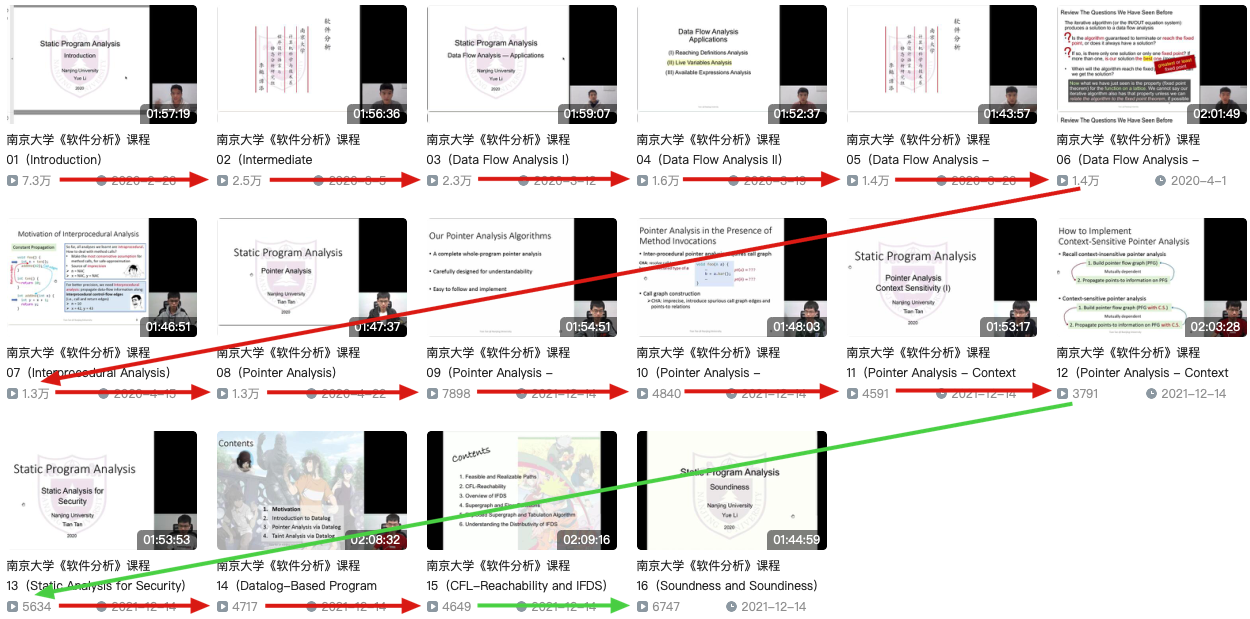## Live Variables Analysis

Live variables analysis tells whether the value of variable v at program point p could be used along some path in CFG starting at p. If so, v is live at p; otherwise, v is dead at p.

1. 首先，变量v在p点之后有被使用。
2. 其次，从p点开始到被使用前，过程中不能出现变量v的重定义。

$$OUT[B] = \bigcup_{S\ a\ successor\ of\ B}IN[S]$$

$$IN[B] = use_{B} \cup (OUT[B] - def_{B})$$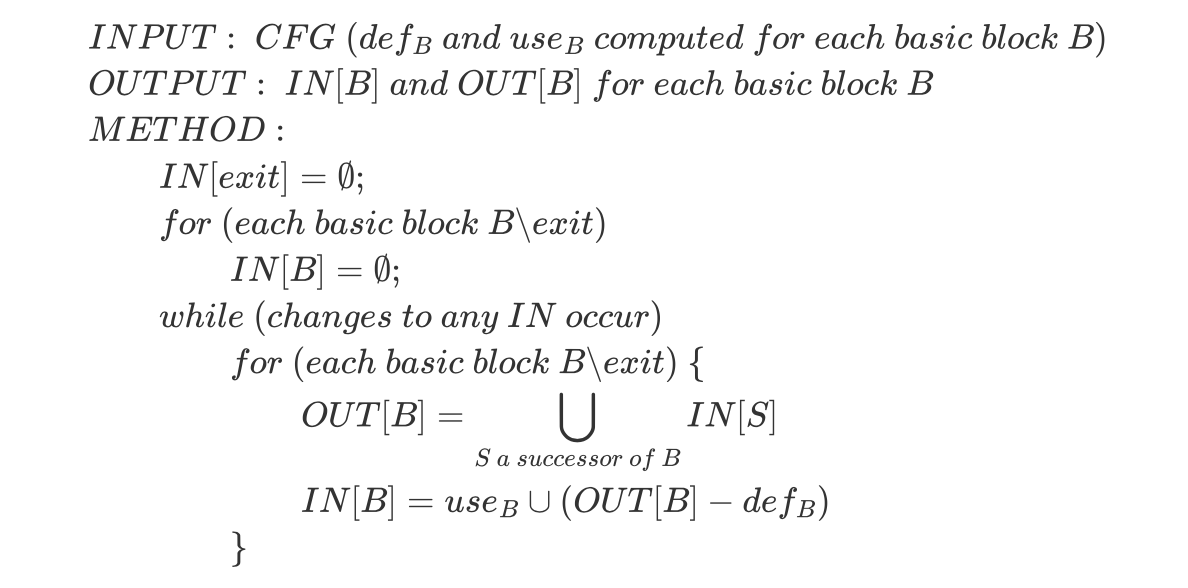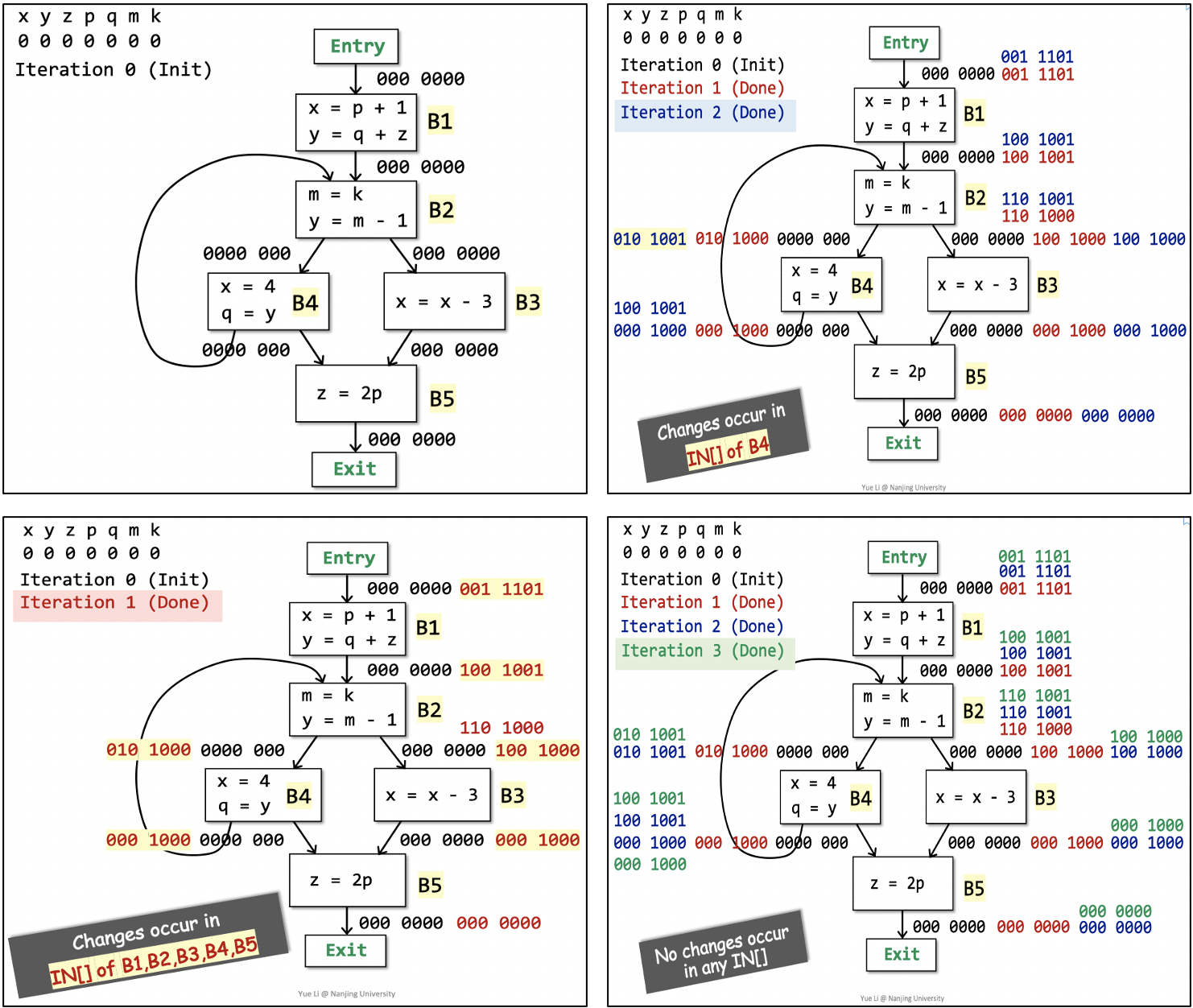## Available Expressions Analysis

An expression ‘x op y’ is available at program point p if: (1) all paths from the entry to p must pass through the evaluation of ‘x op y’, and (2) after the last evaluation of ‘x op y’, there is no redefinition of x or y.

1. 首先，从entry到program point p的路径都要经过x op y的表达式计算。
2. 其次，在最后一处表达式计算之后、p点之前，过程中不能出现变量x或y的重定义。

$$OUT[B] = gen_{B} \cup (IN[B] - kill_{B})$$

$$IN[B] = \bigcap_{P\ a\ predecessor\ of\ B} OUT[P]$$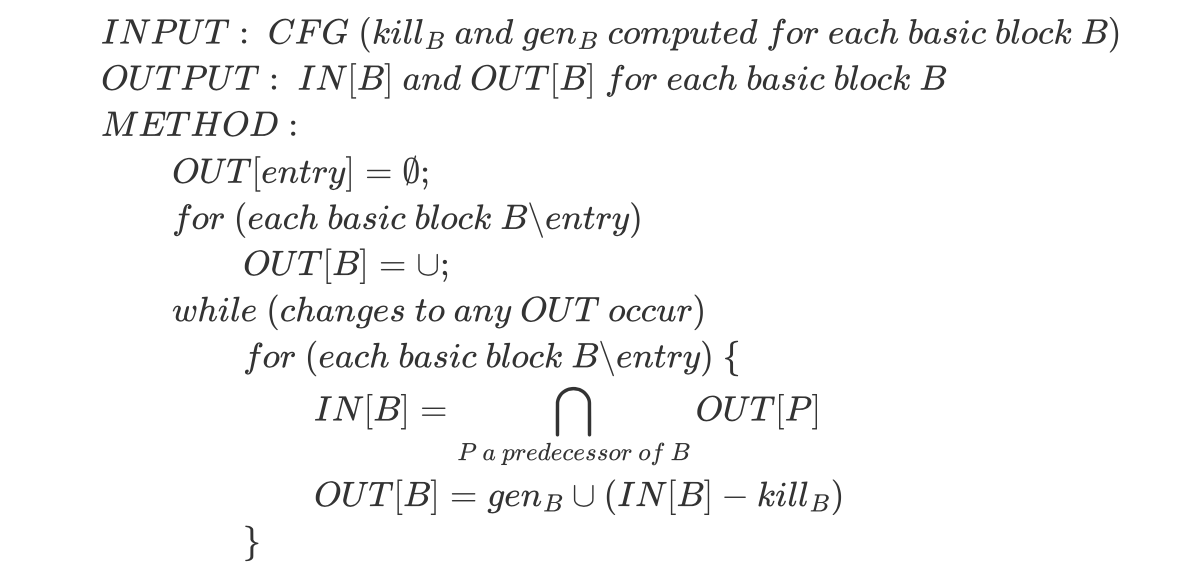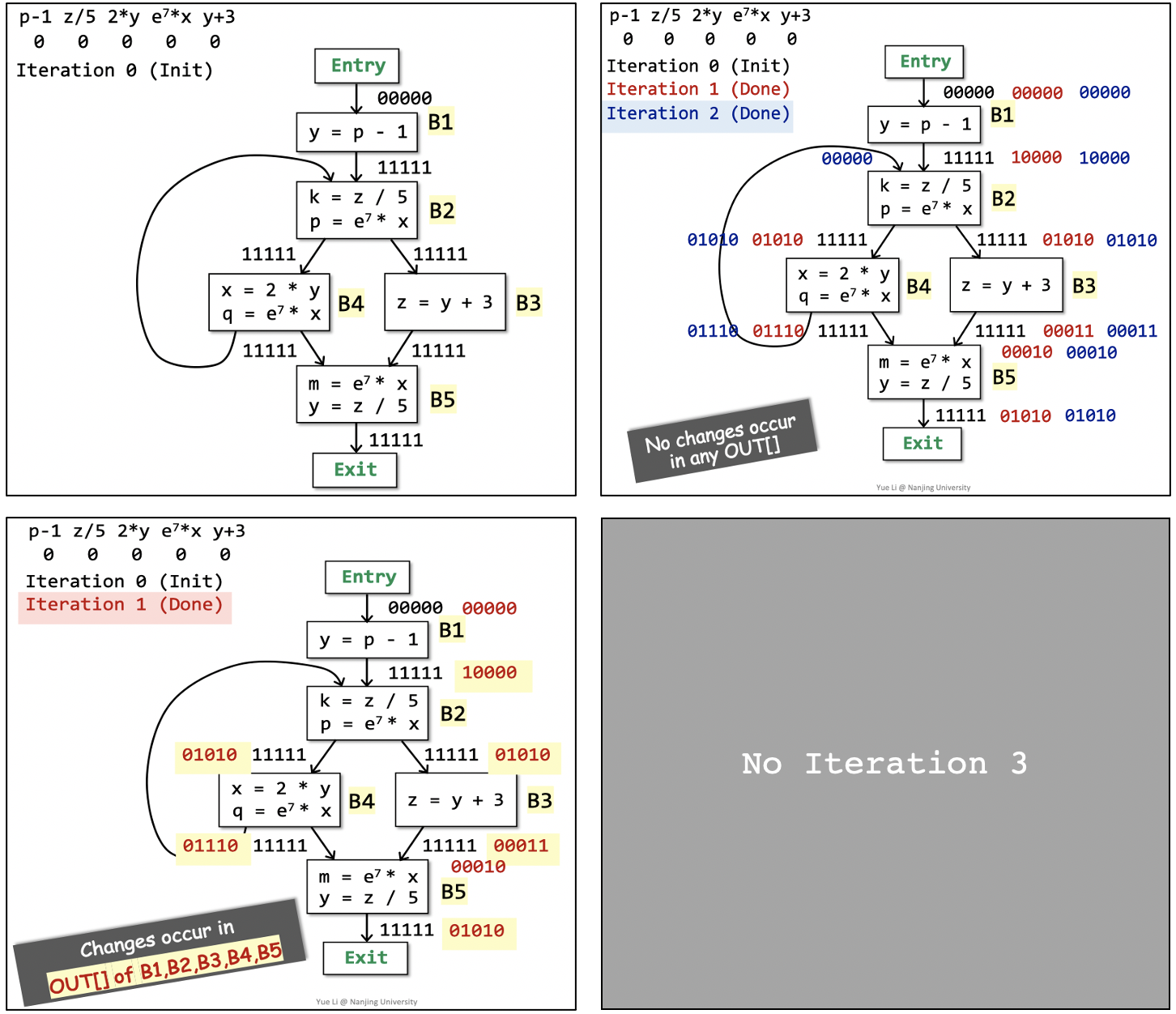## 总结与思考

Reach Definitions Live Variables Available Expressions
Domain set of definitions set of variables set of experssions
Direction forwards backwards forwards
May/Must may may must
Boundary $OUT[entry] = \empty$ $IN[exit] = \empty$ $OUT[entry] = \empty$
Initialization $OUT[B] = \empty$ $IN[B] = \empty$ $OUT[B] = \cup$
Transfer Function $OUT = gen \cup (IN - kill)$ $IN = gen \cup (OUT - kill)$ $OUT = gen \cup (IN - kill)$
Meet $\bigcup$ $\bigcup$ $\bigcap$